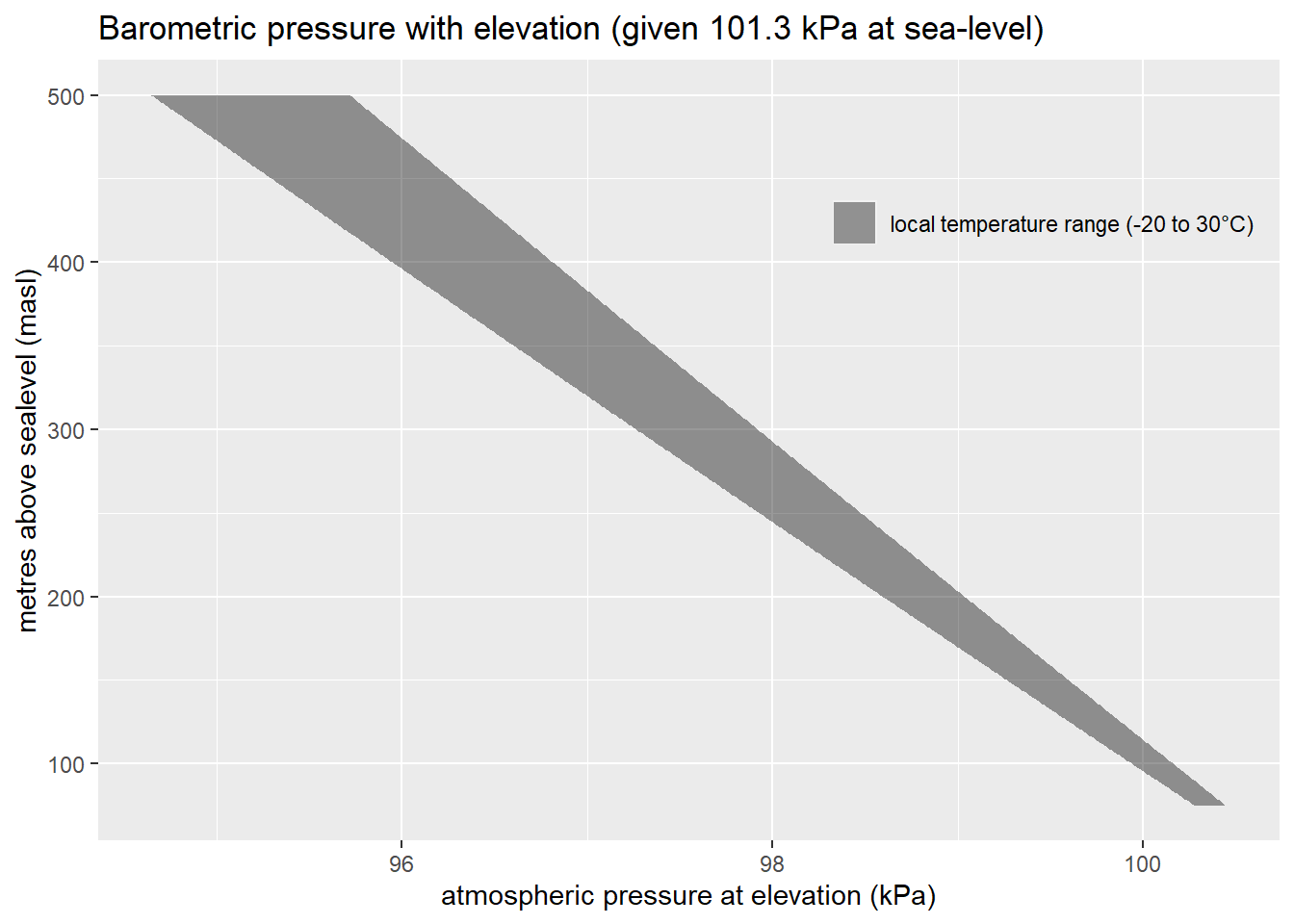## Summary

Spatial Interpolation of hourly measured atmospheric pressure from a set of stations (points) to a regional grid requires correction for elevation. In the modest range of elevations found in the ORMGP jurisdiction (from Lake Ontario surface ~74 metres above sea level (masl) to just below 500 masl), atmospheric pressure can deviate up to 5 kPa. For instance, if the air pressure at Lake Ontario is greater than 100 kPa, given all else the same, the expected range in pressures can be predicted using the the barometric formula:

$P=P_b \cdot \left[\frac{T_b + L_b \cdot (h-h_b)}{T_b}\right]^{\frac{-g_0 \cdot M}{R^\cdot L_b}},$

where:
$$h_b$$: reference elevation (masl)
$$P_b$$: reference pressure at elevation $$h_b$$ (Pa)
$$P$$: pressure at elevation $$h$$ (Pa)
$$T_b$$: reference temperature (K)
$$L_0$$ = -0.0065 K/m temperature lapse rate near earth’s surface
$$R^{*}$$ = 8.3144598 J/(mol·K) universal gas constant
$$g_{0}$$ = 9.80665 m/s² gravitational acceleration
$$M$$ = 0.0289644 kg/mol molar mass of Earth’s air.

With reference temperatures ($$T_b$$) ranging from -20 to 30°C (shaded band), over our elevation range, the pressure deviation can be shown here:### Intent

The initial intent for this interpolated product is to provide an estimate of atmospheric pressure at arbitrary locations within our jurisdiction to test is capability for barometric corrections to groundwater monitors.

## Data Correction

Station data reporting hourly atmospheric pressures $$P$$ are first “corrected” to a reference elevation $$h_b$$, set here as the median elevation of reporting weather stations at a given time step.

def referenceTemperature(ta, elev):
tb = np.median(ta[msk]) + 273.15
zb = np.median(elev[msk])
if np.isnan(tb) or np.isnan(zb):
tb = 288.16 # Sea level standard temperature
zb = 0.0
return tb, zb

With a reference temperature $$T_b$$ set to median measured temperature, pressures are corrected to the reference elevation using the inverse of the barometric equation (note that the constants have been included):

$P_b = P\cdot \left[1-0.0065 \frac{h-h_b}{T_b} \right]^{-5.26}.$

These “reference pressures” are then spatially interpolated to a grid or a set of points and then re-cast to their elevations by:

$P_z = P_b\cdot \left[1-0.0065 \frac{z-h_b}{T_b} \right]^{5.26},$

where $$P_z$$ is the pressure interpolated to cell/point of elevation $$z$$.

## Results

Below is an animation of the hourly interpolation. Locations (points) and the surface are colour-coded the same; discrepancies are made evident where the colours mis-match.